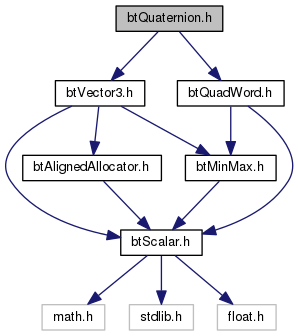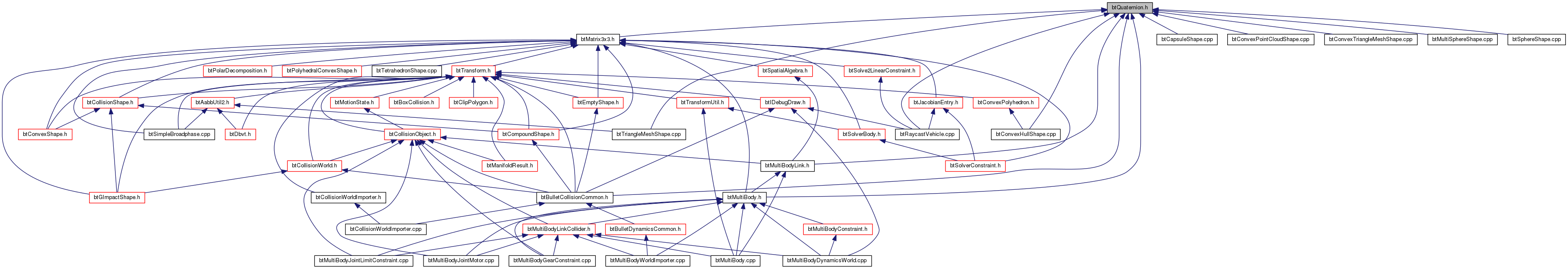Bullet Collision Detection & Physics Library
btQuaternion.h File Reference
`#include "btVector3.h"`
`#include "btQuadWord.h"`
Include dependency graph for btQuaternion.h:This graph shows which files directly or indirectly include this file:Go to the source code of this file.

## Classes

class  btQuaternion
The btQuaternion implements quaternion to perform linear algebra rotations in combination with btMatrix3x3, btVector3 and btTransform. More...

struct  btQuaternionFloatData

struct  btQuaternionDoubleData

## Macros

#define btQuaternionData   btQuaternionFloatData

#define btQuaternionDataName   "btQuaternionFloatData"

## Functions

btQuaternion operator* (const btQuaternion &q1, const btQuaternion &q2)
Return the product of two quaternions. More...

btQuaternion operator* (const btQuaternion &q, const btVector3 &w)

btQuaternion operator* (const btVector3 &w, const btQuaternion &q)

btScalar dot (const btQuaternion &q1, const btQuaternion &q2)
Calculate the dot product between two quaternions. More...

btScalar length (const btQuaternion &q)
Return the length of a quaternion. More...

btScalar btAngle (const btQuaternion &q1, const btQuaternion &q2)
Return the angle between two quaternions. More...

btQuaternion inverse (const btQuaternion &q)
Return the inverse of a quaternion. More...

btQuaternion slerp (const btQuaternion &q1, const btQuaternion &q2, const btScalar &t)
Return the result of spherical linear interpolation betwen two quaternions. More...

btVector3 quatRotate (const btQuaternion &rotation, const btVector3 &v)

btQuaternion shortestArcQuat (const btVector3 &v0, const btVector3 &v1)

btQuaternion shortestArcQuatNormalize2 (btVector3 &v0, btVector3 &v1)

## Macro Definition Documentation

 #define btQuaternionData   btQuaternionFloatData

Definition at line 29 of file btQuaternion.h.

 #define btQuaternionDataName   "btQuaternionFloatData"

Definition at line 30 of file btQuaternion.h.

## Function Documentation

 btScalar btAngle ( const btQuaternion & q1, const btQuaternion & q2 )
inline

Return the angle between two quaternions.

Definition at line 913 of file btQuaternion.h.

 btScalar dot ( const btQuaternion & q1, const btQuaternion & q2 )
inline

Calculate the dot product between two quaternions.

Definition at line 898 of file btQuaternion.h.

 btQuaternion inverse ( const btQuaternion & q )
inline

Return the inverse of a quaternion.

Definition at line 920 of file btQuaternion.h.

 btScalar length ( const btQuaternion & q )
inline

Return the length of a quaternion.

Definition at line 906 of file btQuaternion.h.

 btQuaternion operator* ( const btQuaternion & q1, const btQuaternion & q2 )
inline

Return the product of two quaternions.

Definition at line 643 of file btQuaternion.h.

 btQuaternion operator* ( const btQuaternion & q, const btVector3 & w )
inline

Definition at line 731 of file btQuaternion.h.

 btQuaternion operator* ( const btVector3 & w, const btQuaternion & q )
inline

Definition at line 814 of file btQuaternion.h.

 btVector3 quatRotate ( const btQuaternion & rotation, const btVector3 & v )
inline

Definition at line 937 of file btQuaternion.h.

 btQuaternion shortestArcQuat ( const btVector3 & v0, const btVector3 & v1 )
inline

Definition at line 951 of file btQuaternion.h.

 btQuaternion shortestArcQuatNormalize2 ( btVector3 & v0, btVector3 & v1 )
inline

Definition at line 970 of file btQuaternion.h.

 btQuaternion slerp ( const btQuaternion & q1, const btQuaternion & q2, const btScalar & t )
inline

Return the result of spherical linear interpolation betwen two quaternions.

Parameters
 q1 The first quaternion q2 The second quaternion t The ration between q1 and q2. t = 0 return q1, t=1 returns q2 Slerp assumes constant velocity between positions.

Definition at line 931 of file btQuaternion.h.